Next: 4.3 Capacitance-Voltage Characteristics Up: 4. Characterization of Interfaces Previous: 4.1 Charge Pumping Method

# 4.2 DCIV Method

Another experimental method for the investigation of the interface quality is the direct-current current-voltage (DCIV) method. It can be used to determine both the interface trap density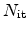and the amount of fixed oxide charges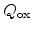.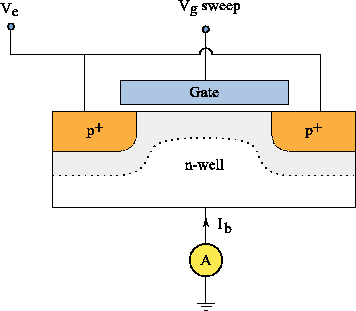The experimental set-up is illustrated in Figure 4.10. The source and drain to substrate diodes are slightly forward biased, typically with around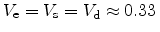V. The gate bias is swept from inversion to slight accumulation. During this sweep the substrate current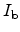is measured. The substrate current originates from recombination of carriers at the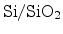interface.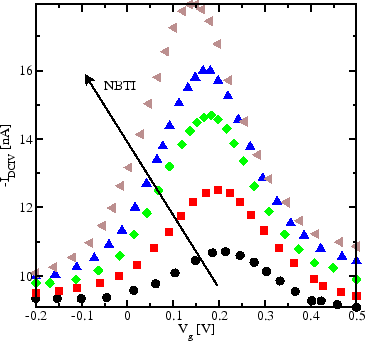For each degradation level there is a clear peak of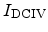at a certain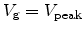, as shown in Figure 4.11. The peak height above the base line,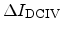is approximately proportional to the effective interface trap densityas [46,47]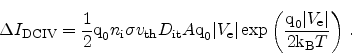(4.7)

Here,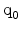is the elementary charge,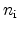the intrinsic carrier concentration,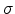the geometric mean of the capture cross sections for electrons and holes, equal to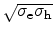,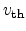the thermal velocity,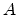the effective gate area,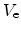the forward bias applied to the source-substrate and drain-substrate junctions,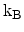Boltzmann's constant, and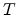the temperature.

To determine, the oxide charge density, the peak position of the DCIV curve can be used. As described in Section 2.2.1, the peak position is directly proportional to the amount of oxide charges and can be calculated using Equation 2.31.Next: 4.3 Capacitance-Voltage Characteristics Up: 4. Characterization of Interfaces Previous: 4.1 Charge Pumping Method

R. Entner: Modeling and Simulation of Negative Bias Temperature Instability